• 数据结构与算法课设
2022-07-06 19:21:49

# 江苏大学公交系统(数据结构与算法课设版C++)

目录

江苏大学公交系统(数据结构与算法课设版C++)

(1)题目要求

(2)功能要求

(3) 结构

(4) 不足

附录

## (1)题目要求

为2022级新生定制一个线路问询交互系统，依据参考因素，例如：换乘路线的路径最短、耗费时间最短、所需车资最少等，经过分析处理得到可达目的站点换乘次数最少的乘车方案，具体可分为：

(1)零次换乘 起始站点和目的站点之间存在可直达的公交线路，即出行无需转乘就可以直接到达目的站点，这也是较为理想的方案。

(2)一次换乘 起始站点和目的站点之间没有公交车直接往返，即两站点之间不存在可直达的公交线路，则出行居民需要在途经的某个站点下车，然后转乘另一线路公交车才能达到目的站点。

(3)步行 基于地图用户可以按照最近距离步行前往某个地点。

## (2)功能要求

1. 初始化系统：

基本信息初始化，包括公交线路编号、站点名称、站点之间票价、耗费时间、发车时间等（根据自己设计可以增加相关公交车信息，）；

基本要求：将公交线路的基本信息存于文件，每次运行程序时，通过文件对基本信息初始化；

1. 公交信息维护：

由于修路等城市规划变动的原因，公交线路可能会有微调，例如需要增加、删除或者修改部分站点的信息；修改之后的课程信息写回文件，便于下一次初始化；

1. 查询功能：

从键盘输入编号，给出具体行车路线、行车时间等；

从键盘输入始发站和终点站，完成公交线路换乘查询（零次，1次）

最短线路规划：从五棵松至校内其余地点的最短路线

1. 用户角色：

分为管理员和学生，管理员可以进行初始化、公交信息维护和查询功能；游客只可以使用查询功能。

## (3) 结构

程序内使用邻接矩阵存储校内地图，自定义结构体存储校内公交信息。

使用文件读取获取具体线路以及公交信息。

最短路线使用Dijkstra完成计算。

## (4) 不足

未完全实现最短路线查询功能，未实现步行+公交换乘，如读者对此方面有想法可以私信与我联系交流一下具体实现方法。本人只是一名学习者，程序中可能存在诸多问题，如果读者有兴趣仔细阅读改正，可以在评论区交流一下，不喜勿喷，谢谢！

## 附录

其中还有数据结构与算法的一些代码包含(C语言和C++)数据结构
更多相关内容
• 广东工业大学计算机数据结构与算法课设，包括伸展树等
• 数据结构与算法课设——医院候诊管理系统.docx数据结构与算法课设——医院候诊管理系统.docx数据结构与算法课设——医院候诊管理系统.docx数据结构与算法课设——医院候诊管理系统.docx数据结构与算法课设——医院...
• PAGE 26 任 务 书 题目通讯录管理系统 设计内容及要求 1.课程设计任务内容 制作一个简易的通讯录管理系统根据不同的选项进行添加修改删除遍历联系人这四个操作且对于联系人有亲人朋友同事其他四个分组 2....
• 学生成绩管理系统源代码(数据结构与算法课设)C语言版.pdf
• 个人资料整理 仅限学习使用 2018 年秋季学期数据结构与算法课程设计题目 1. 扫雷问题 有些个人计算机会带有一个名为 Minesweeper 的游戏该游戏界 面是一个网格网格中的有些方块是雷编写一个程序以读取文件该文件 中...
• 学生成绩管理系统论文加源代码数据结构与算法课设C语言.doc
• 学生成绩管理系统源代码_(数据结构与算法课设)C语言版.doc
• 数据结构选题：排序算法比较课程设计答辩问题描述：本次课设小组共选择 5 大类算法，进行比较。设计过程中难点主要在于理解算法思想，代码编写给各个算法随机排序较多的数以达到计时比较目的。解决方案：在算法...
• 毕业,课程,设计,数据结构,算法,斗地主,洗牌毕业,课程,设计,数据结构,算法,斗地主,洗牌这是从斗地主源程序选取的五个功能模块，分别为洗牌模块，对玩家的初始化，游戏初始化，补全手牌信息模块，获取缓冲区牌类型模块...

毕业,课程,设计,数据结构,算法,斗地主,洗牌毕业,课程,设计,数据结构,算法,斗地主,洗牌

这是从斗地主源程序选取的五个功能模块，分别为洗牌模块，对玩家的初始化，游戏初始化，

补全手牌信息模块，获取缓冲区牌类型模块。

一、我的程序实现了哪些功能:

(1)洗牌功能

static deck* deck_init(){

int i,j;

srand(time(0));

deck *p_deck=(deck*)malloc(sizeof(deck));

if(!p_deck){

printf("分配内存失败\n");

return NULL;

}

for(i=1;i<=54;i++){

p_deck->arr[i]=rand()%54;

for(j=1;j

if(p_deck->arr[i]==p_deck->arr[j]){

i--;

break;

}

}

}

p_deck->top=54;

return p_deck;

}

(2)初始化玩家(洗牌，id，身份 手牌,总数)功能

static void player_init(){

int i,j;

for(j=0;j<3;j++){

for(i=1;i<=20;i++){

player[j].handcard[i].number=100;

player[j].handcard[i].level =0;

}

}

deck *p=deck_init();

if(!p){

printf("没有牌堆\n");

return ;

}

int which=0;

which=rand()%3;

game.who=which;

for(i=0;i<3;i++){

player[i].id=i;

if(i==which){//地主

player[i].status=1;

for(j=1;j<=20;j++){

player[i].handcard[j].number=p->arr[(p->top)--];

}

player[i].size=20;

}

else{//农民

player[i].status=0;

for(j=1;j<=17;j++){

player[i].handcard[j].number=p->arr[(p->top)--];

}

player[i].size=17;

}

}

free(p);

p=NULL;

}

(3)手牌信息补完功能

static void handcard_init(){

int i,j;

for(i=0;i<3;i++){

for(j=1;j<=20;j++){

int number=player[i].handcard[j].number;

int *p_level=&(player[i].handcard[j].level);

char **pp_flower=&(player[i].handcard[j].flower);

char *p_point=&(player[i].handcard[j].point);

if(number>=0&&number<=51){

*p_level=number/4+1;

*p_point=point[number/4+1];

*pp_flower=farr[number%4];

}

else if(number==52){

*p_level=14;

*p_point='w';

*pp_flower="小王";

}

else if(number==53){

*p_level=15;

*p_point='W';

*pp_flower="大王";

}

else {

*p_level=0;

*p_point=' ';

*pp_flower=" ";

}

}

}

}

(4)打印当前玩家手牌功能

void print(){

int i,j;

for(i=0;i<3;i++){

if (

展开全文• 并且实现地点的查询，增加，删除以及更新操作，并且可视化整张地图，基于Dijkstra算法实现最短路径的查找规划，完成地点无向图的构建，采用邻接矩阵的存储方式并实现以下功能： 1、输出所有地点及其介绍 2、查询...

目录

一、课设目的

二、课设原理

三、课设内容

四、基本要求

五、设计源程序

六、测试结果

七、总结心得

八、致谢&参考文献

# 一、课设目的

1.综合应用已学过的数据结构与算法知识，实现课程设计。

2.加深对课程内容的理解，提高软件设计、编写及调试程序的能力。

3.提高对问题的抽象能力和算法的运用能力。

4.进一步理解最短路径算法的原理及并综合编程实践。

# 二、课设原理

定义交通图的存储结构。邻接矩阵是表示图形中顶点之间相邻关系的矩阵。设G=(V,E)是具有n个顶点的图，则G的邻接矩阵是具有如下定义的n阶方阵。

注：一个图的邻接矩阵表示是唯一的，其表示需要用一个二维数组存储顶点之间相邻关系的邻接矩阵并且还需要用一个具有n个元素的一维数组来存储顶点信息（下标为i的元素存储顶点的信息）。

邻接矩阵的存储结构：

typedef struct
{
char name;
char intro;
int  x, y;
}SITE;
typedef struct
{
}MAP;


最短路径问题：已知无向图(带权)，期望找出从某个源点S∈V到G中其余各顶点的最短路径。Dijkstra算法即按路径长度递增产生诸顶点的最短路径算法。

算法原理：设有向图G=(V,E)，其中V={1,2，……n}，cost是表示G的邻接矩阵，length [i][j]表示有向边<i,j>的权。若不存在有向边<i,j>，则length [i][j] 的权为无穷大（这里取值为32767）。设S是一个集合，集合中一个元素表示一个顶点，从源点到这些顶点的最短距离已经求出。设顶点V1为源点，集合S的初态只包含顶点V1。数组dist记录从源点到其它各顶点当前的最短距离，其初值为dist[i]= length [i][j]，i=2，……n。从S之外的顶点集合V-S中选出一个顶点w，使dist[w] 的值最小。于是从源点到达w只通过S中的顶点，把w加入集合S中，调整dist中记录的从源点到V-S中每个顶点v的距离：从原来的dist[v]和dist[w]+ length [w][v]中选择较小的值作为新的dist[v]。重复上述过程，直到S中包含V中其余顶点的最短路径。

最终结果是：S记录了从源点到该顶点存在最短路径的顶点集合，数组dist记录了从源点到V中其余各顶点之间的最短路径，path是最短路径的路径数组，其中path[i]表示从源点到顶点i之间的最短路径的前驱顶点。

# 三、课设内容

一张青岛地图包括n个地点，假设地点间有m条路径，每条路径的长度已知。给定地图的一个起点和终点，利用Dijsktra算法求出起点到终点之间的最短路径。并且实现地点的查询，增加，删除以及更新操作，并且可视化整张地图，基于Dijkstra算法实现最短路径的查找与规划，完成地点无向图的构建，采用邻接矩阵的存储方式并实现以下功能：

1、输出所有地点及其介绍

2、查询某一个地点及其介绍

3、增加一个地点

4、删除一个地点

5、更新一个地点

6、增加一条路

7、删除一条路

8、更新一条路

9、查询某一地点到其他所有地点的最短路径

10、查询某两个地点之间的最短路径

11、输出地图

# 四、基本要求

1.界面友好：有合理的中文提示，每个功能可以设立菜单，根据提示，可以完成相关的功能。出现非法输入，会给出异常提示。

2.物理存储：相关数据要求存储在某一数据结构中，在程序中完成数据结构的定义与说明。

3.逻辑结构：根据问题的要求，采用线性或非线性结构。实验分析中考虑数据量问题。

# 五、设计源程序

#include <stdio.h>
#include <stdlib.h>
#include <conio.h>
#include <string.h>
#include <graphics.h>
using namespace std;

#define INF 99999

typedef struct
{
char name;
char intro;
int  x, y;
}SITE;

typedef struct
{
}MAP;

MAP M;
int N = 0;
path p;
dist d;

void init()
{
int i, j;
strcpy(M.site.name, "胶东机场");
strcpy(M.site.intro, "青岛胶东国际机场（Qingdao Jiaodong International Airport），位于中国山东省青岛市胶州市胶东街道前店口村");
strcpy(M.site.name, "红岛火车站");
strcpy(M.site.intro, "山东省青岛市城阳区红岛经济区河套街道汇海路南侧");
strcpy(M.site.name, "流亭");
strcpy(M.site.intro, "青岛流亭国际机场（已关闭）");
strcpy(M.site.name, "青岛站");
strcpy(M.site.intro, "山东省青岛市市南区泰安路2号");
strcpy(M.site.name, "青岛北站");
strcpy(M.site.intro, "山东省青岛市李沧区静乐路1号");
strcpy(M.site.name, "李村");
strcpy(M.site.intro, "李村隶属于山东省青岛市李沧区");
strcpy(M.site.name, "五四广场");
strcpy(M.site.intro, "五四广场（May Fourth Square），位于山东省青岛市市南区东海西路");
strcpy(M.site.name, "中山公园");
strcpy(M.site.intro, "青岛最大的综合性公园");
strcpy(M.site.name, "麦岛");
strcpy(M.site.intro, "麦岛是山东省青岛市区沿岸第一大岛");
strcpy(M.site.name, "中心医院");
strcpy(M.site.intro, "青岛市中心医院，青岛市中心医院位于山东省青岛市四方区四流南路127号");
strcpy(M.site.name, "QAU");
strcpy(M.site.intro, "青岛农业大学（Qingdao Agricultural University），位于山东省青岛市");
strcpy(M.site.name, "台东");
strcpy(M.site.intro, "台东区是山东省青岛市原辖区，地名沿用自原区划台东镇。");
strcpy(M.site.name, "遵义路");
strcpy(M.site.intro, "山东省青岛市李沧区");
strcpy(M.site.name, "鳌山卫");
strcpy(M.site.intro, "鳌山卫镇位于即墨市东隅,镇机关驻地距即墨市政府所在地20公里。");
strcpy(M.site.name, "苗岭路");
strcpy(M.site.intro, "苗岭路站（Miaoling Rd Station），位于中国山东省青岛市深圳路与苗岭路交叉口南侧");
strcpy(M.site.name, "SDU");
strcpy(M.site.intro, "山东大学（青岛）位于山东省青岛市即墨区“蓝色硅谷”核心区");
strcpy(M.site.name, "OUC");
strcpy(M.site.intro, "中国海洋大学（Ocean University of China，OUC），位于山东省青岛市");
for (i = 1; i <= ALLNameNum; i++)
{
for (j = 1; j <= ALLNameNum; j++)
{
M.length[i][j] = INF;
}
}
for (i = 1; i <= ALLNameNum; i++)
M.length[i][i] = 0;
M.length = M.length = 30;
M.length = M.length = 30;
M.length = M.length = 90;
M.length = M.length = 70;
M.length = M.length = 50;
M.length = M.length = 50;
M.length = M.length = 50;
M.length = M.length = 100;
M.length = M.length = 30;
M.length = M.length = 110;
M.length = M.length = 20;
M.length = M.length = 30;
M.length = M.length = 30;
M.length = M.length = 30;
M.length = M.length = 30;
M.length = M.length = 60;
M.length = M.length = 40;
M.length = M.length = 40;
M.length = M.length = 100;
M.length = M.length = 50;
M.length = M.length = 60;
M.length = M.length = 30;
M.length = M.length = 170;
M.length = M.length = 70;
M.length = M.length = 30;
M.length = M.length = 50;
M.length = M.length = 50;
M.length = M.length = 20;
M.length = M.length = 30;
N = 17;
M.site.x = 50;
M.site.y = 25;
M.site.x = 150;
M.site.y = 125;
M.site.x = 250;
M.site.y = 25;
M.site.x = 150;
M.site.y = 475;
M.site.x = 350;
M.site.y = 175;
M.site.x = 475;
M.site.y = 50;
M.site.x = 475;
M.site.y = 475;
M.site.x = 575;
M.site.y = 650;
M.site.x = 675;
M.site.y = 525;
M.site.x = 575;
M.site.y = 150;
M.site.x = 750;
M.site.y = 225;
M.site.x = 575;
M.site.y = 525;
M.site.x = 575;
M.site.y = 225;
M.site.x = 1075;
M.site.y = 25;
M.site.x = 1000;
M.site.y = 375;
M.site.x = 1100;
M.site.y = 225;
M.site.x = 1175;
M.site.y = 325;
}

void queryAllSite()
{
int t;
printf("所有地点如下：\n");
for (t = 1; t <= N; t++)
{
printf("编号：%d\n   地点：%s\n   介绍：%s\n", t, M.site[t].name, M.site[t].intro);
}
printf("\n任意键返回");
getch();
}

{
char s1, s2;    //地点名称；地点介绍
int t, i, q, p, x, y;       //t为与该地点共有几个地点相连；i为应第几个地点与之相连；
//q为与之相连的地点；p为这个地点和新地点的距离
//x为此地点位置的x值；y为此地点位置的y值
N++;
printf("请输入该地点的名称：");
scanf("%s", &s1);
printf("请输入该地点的介绍：");
scanf("%s", &s2);
printf("请输入该地点的位置的x值：");
scanf("%d", &x);
printf("请输入该地点的位置的y值：");
scanf("%d", &y);
strcpy(M.site[N].name, s1);
strcpy(M.site[N].intro, s2);
M.site[N].x = x;
M.site[N].y = y;
printf("请输入共有几个地点与此地点相通：");
scanf("%d", &t);
for (i = 1; i <= t; i++)
{
printf("第%d个地点与之相通；", i);
scanf("%d", &q);
printf("他们之间的距离为：");
scanf("%d", &p);
M.length[N][q] = M.length[q][N] = p;
}
printf("\n按任意键返回！");
getch();
}

void delSite()
{
printf("请输入要删除的地点序号数");
int t, p, Q;
scanf("%d", &p);
Q = p;
if (p <= N)
{//N节点数量
for (t = 1; t <= N; t++)
for (p = p; p <= N; p++)
{
M.length[t][p] = M.length[t][p + 1];
M.length[p][t] = M.length[p + 1][t];
}
for (Q; Q < N; Q++)
M.site[Q] = M.site[Q + 1];
printf("删除成功");
}
else
printf("删除失败");
N--;
printf("\n按任意键返回！");
getch();
}

void reSite()
{
int t;
char s1, s2;
printf("请输入要更新的地点编号：");
scanf("%d", &t);
if (t > N)
printf("此地点不存在，更新失败");
else
{
printf("请输入地点名称：");
scanf("%s", &s1);
printf("请输入地点介绍：");
scanf("%s", &s2);
strcpy(M.site[t].name, s1);
strcpy(M.site[t].intro, s2);
printf("更新完成！");
}
printf("\n按任意键返回！");
getch();
}

{
int a, b, c;
printf("请问要在那两个地点之间增加一条路\n");
printf("请输入第一个地点编号：");
scanf("%d", &a);
printf("请输入第二个地点编号：");
scanf("%d", &b);
printf("请输入这条路的长度：");
scanf("%d", &c);
if (a > N || b > N)
printf("地点超出了已有地点范围，路径增加失败！");
else
{
M.length[a][b] = M.length[b][a] = c;
printf("路径增加成功！");
}
printf("\n按任意键返回！");
getch();
}

void delpath()
{
int a, b;
printf("删除路径的编号\n");
printf("请输入第一个地点编号：");
scanf("%d", &a);
printf("请输入第二个地点编号：");
scanf("%d", &b);
if (a > N || b > N)
printf("越界，路径删除失败！");
else
{
M.length[a][b] = M.length[b][a] = INF;
printf("路径删除成功！");
}
printf("\n按任意键返回！");
getch();
}

void repath()
{
int a, b, c;
printf("请问要更新那两个地点之间的路\n");
printf("请输入第一个地点编号：");
scanf("%d", &a);
printf("请输入第二个地点编号：");
scanf("%d", &b);
printf("请输入更新后的长度：");
scanf("%d", &c);
if (a > N || b > N)
printf("这条路不存在，路径更新失败！");
else
{
M.length[a][b] = M.length[b][a] = c;
printf("路径更新成功！");
}
printf("\n按任意键返回！");
getch();
}

void querySite()
{
int a;
printf("请问您要查询的地点编号是：");
scanf("%d", &a);
if (a > N)
printf("查询的地点不存在，查询失败！");
else
printf("编号：%d\n   地点：%s\n   介绍：%s\n", a, M.site[a].name, M.site[a].intro);
printf("\n按任意键返回！");
getch();
}

void dijkstraAllSite()
{
int v0;
printf("请输入查询的地点：");
scanf("%d", &v0);
//v表示上一个节点，k表示遍历节点
int i, k, j, v, min, x;
for (v = 1; v <= N; v++)
{
flag[v] = FALSE;
d[v] = M.length[v0][v];
if (d[v] < INF && d[v] != 0)
p[v] = v0;
else
p[v] = -1;
}
flag[v0] = TRUE;
d[v0] = 0;//原点距离
for (i = 2; i <= N; i++)
{
min = INF;
for (k = 1; k <= N; ++k)
if (!flag[k] && d[k] < min)
{//没有被查询过并且距离小于最小值则更新
v = k;
min = d[k];
}

if (min == INF)
return;
flag[v] = TRUE;
for (k = 1; k <= N; ++k)
if (!flag[k] && (min + M.length[v][k] < d[k]))
{
d[k] = min + M.length[v][k];//到k的距离
p[k] = v;//k节点的上一个节点是v
}
}
}

void printAllSite()
{
int st[ALLNameNum], i, pre, top = -1;
for (i = 1; i <= N; i++)
{
printf("\n到达地点%2d的总距离为: %5d , 经过路径为:", i, d[i]);
st[++top] = i;
pre = p[i];
while (pre != -1)
{
st[++top] = pre;
pre = p[pre];
}
while (top > 0)
{
printf("%d", st[top--]);
if (top > 0)
printf("--->");
}
}
getch();
}

void dijkstraTwoSite()
{
int v0;
printf("请输入起始地点：");
scanf("%d", &v0);
int i, k, j, v, min, x;
for (v = 1; v <= N; v++)
{
flag[v] = FALSE;
d[v] = M.length[v0][v];
if (d[v] < INF && d[v] != 0)
p[v] = v0;
else
p[v] = -1;
}
flag[v0] = TRUE;
d[v0] = 0;
for (i = 2; i <= N; i++)
{
min = INF;
for (k = 1; k <= N; ++k)
if (!flag[k] && d[k] < min)
{
v = k;
min = d[k];
}

if (min == INF)
return;
flag[v] = TRUE;
for (k = 1; k <= N; ++k)
if (!flag[k] && (min + M.length[v][k] < d[k]))
{
d[k] = min + M.length[v][k];
p[k] = v;
}
}
}

void printTwoSite()
{
int y;
printf("\n请输入目的地点：");
scanf("%d", &y);
int st[ALLNameNum], i, pre, top = -1;
for (i = 1; i <= N; i++)
{
if (i == y)
printf("\n总距离是: %5d , 经过路径为:", d[i]);
st[++top] = i;
pre = p[i];
while (pre != -1)
{
st[++top] = pre;
pre = p[pre];
}
while (top > 0)
{
if (i == y)
{
printf("%d", st[top--]);
if (top > 0)
printf("--->");
}
else
top--;
}
}
getch();
}

void printGraph()
{
int i, j;
char s;
strcpy(s, "交通地图");
initgraph(1200, 800);
for (i = 1; i <= N; i++)
{
setfillcolor(RGB(225, 236, 0));
fillcircle(M.site[i].x, M.site[i].y, 20);
outtextxy(M.site[i].x, M.site[i].y, LPCTSTR(M.site[i].name));
}
for (i = 1; i <= N; i++)
for (j = 1; j <= N; j++)
{
if (M.length[i][j] > 0 && M.length[i][j] < 1000)
line(M.site[i].x, M.site[i].y, M.site[j].x, M.site[j].y);
}
outtextxy(900, 650, LPCTSTR(s));
getch();
closegraph();
}

main()
{
init();
int x;
while (1)
{
printf("**********************************************************************\n");
printf("*                 欢迎使用基于交通路线的规划系统                     *\n");
printf("**********************************************************************\n");
printf("\n                     0、退出程序            ");
printf("\n                     1、输出所有地点及其介绍");
printf("\n                     2、查询某一个地点及其介绍");
printf("\n                     3、增加一个地点");
printf("\n                     4、删除一个地点");
printf("\n                     5、更新一个地点");
printf("\n                     6、增加一条路");
printf("\n                     7、删除一条路");
printf("\n                     8、更新一条路");
printf("\n                     9、查询某一地点到其他所有地点的最短路径");
printf("\n                    10、查询某两个地点之间的最短路径   ");
printf("\n                    11、输出地图                       目前地点数：%d\n", N);
printf("请输入选项：");
scanf("%d", &x);
if (x == 0)
break;
else
switch (x)
{
case 1:queryAllSite(); break;
case 2:querySite(); break;
case 4:delSite(); break;
case 5:reSite(); break;
case 7:delpath(); break;
case 8:repath(); break;
case 9:dijkstraAllSite(); printAllSite(); break;
case 10:dijkstraAllSite(); printTwoSite(); break;
case 11:printGraph(); break;
}
system("cls");
}
}


# 六、测试结果

运行程序如下所示：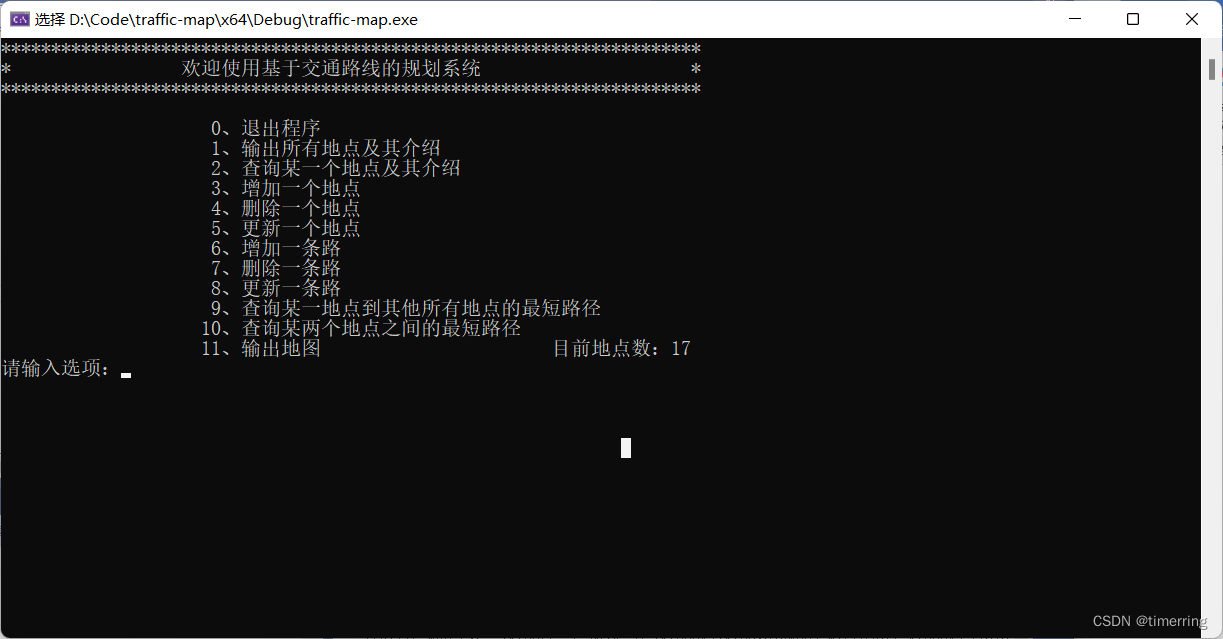分别进行功能测试：

1.输出所有地点及其介绍，结果如下所示：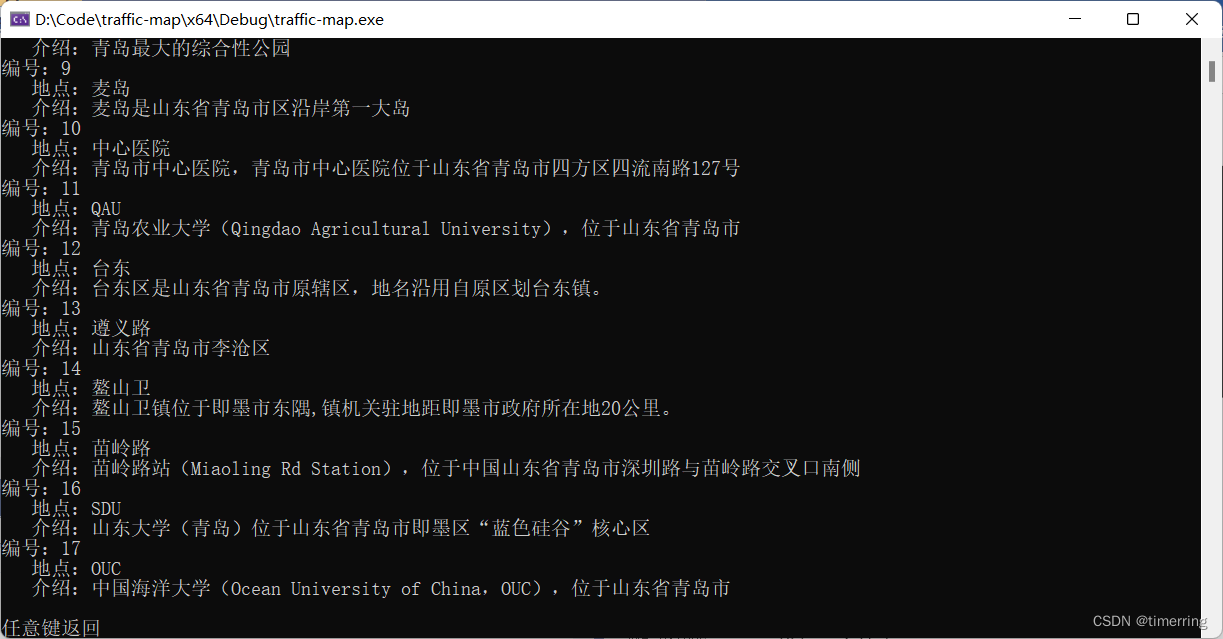2.查询某一个地点及其介绍，结果如下所示：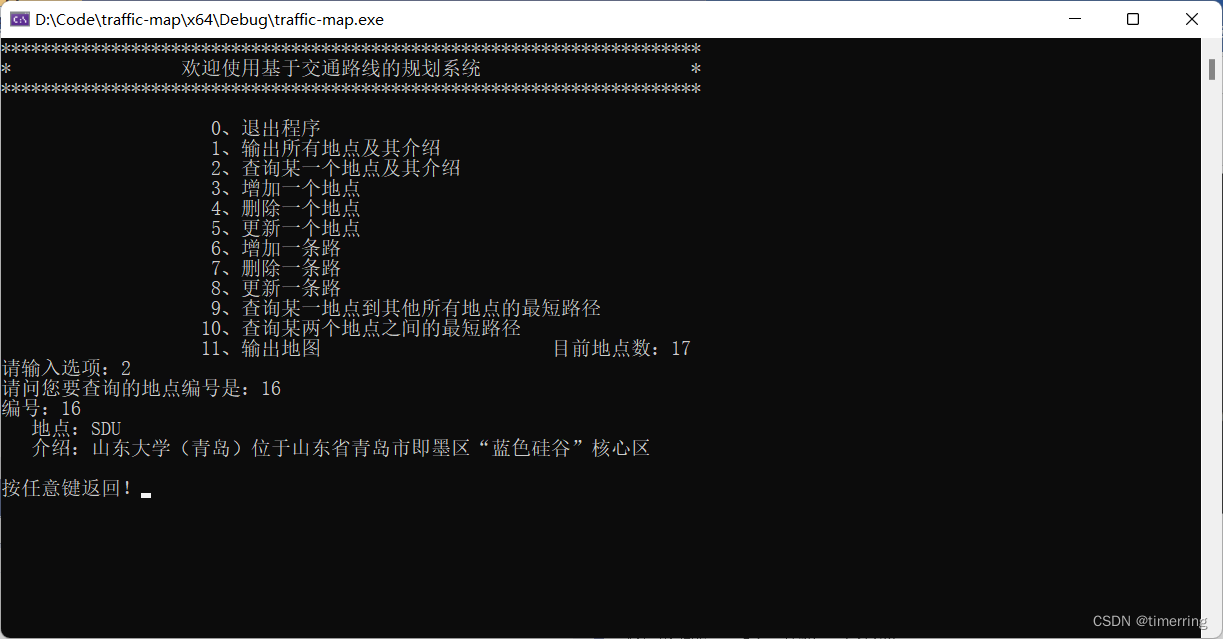3.增加一个地点，结果如下所示：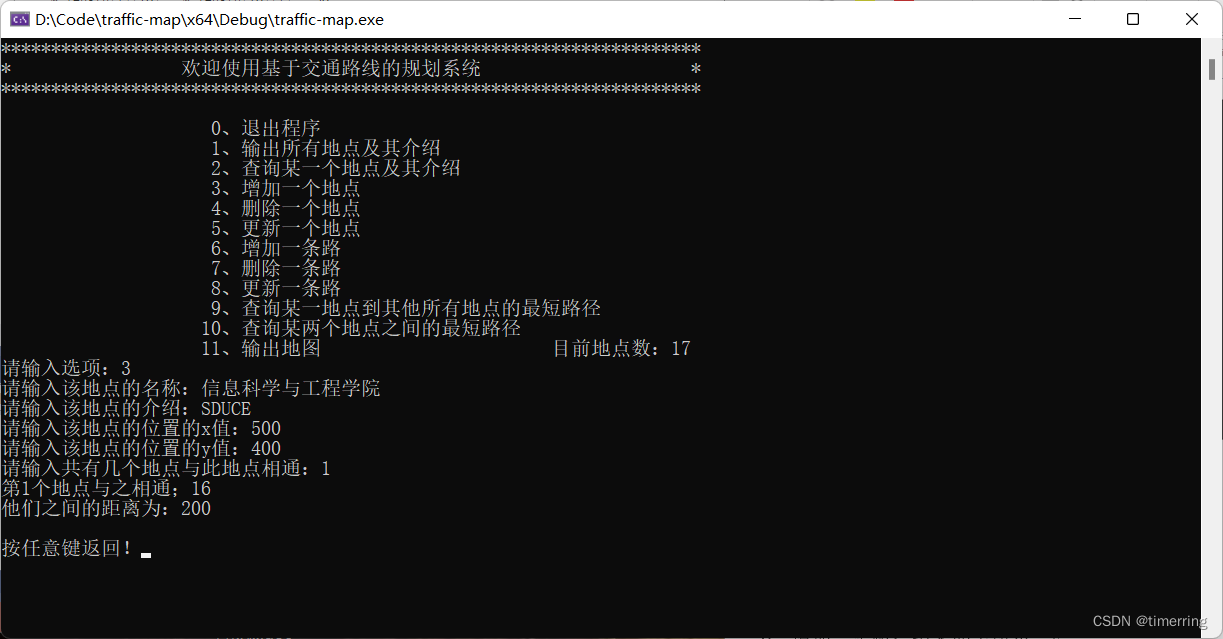这里添加样例为：信息科学与工程学院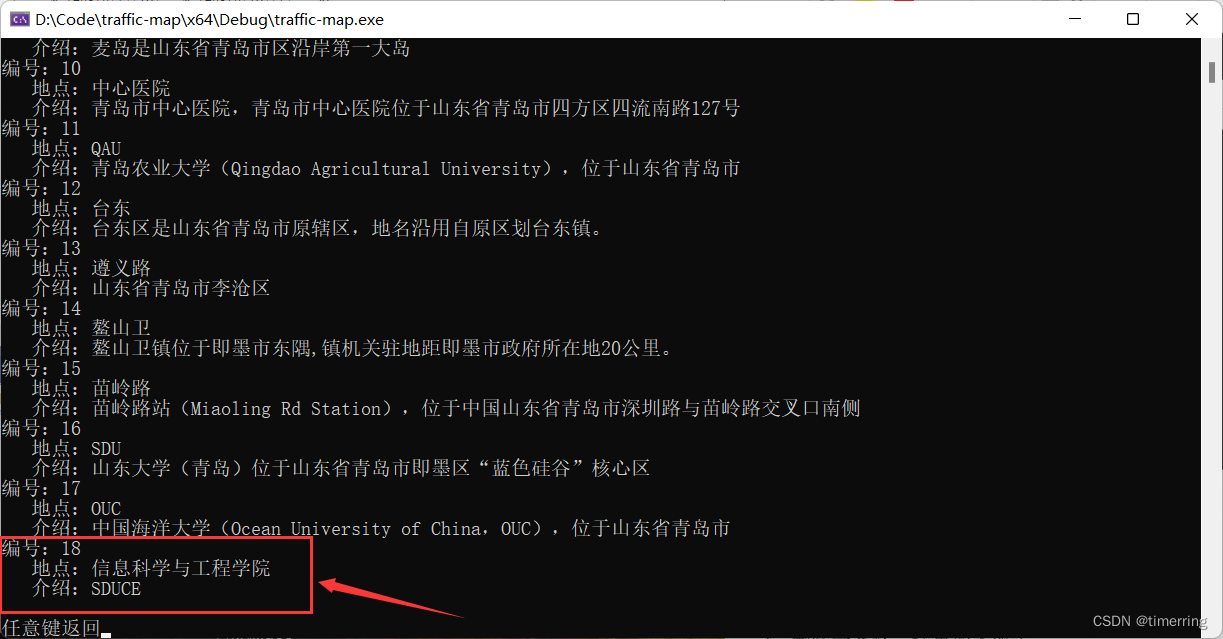4.删除一个地点，结果如下所示：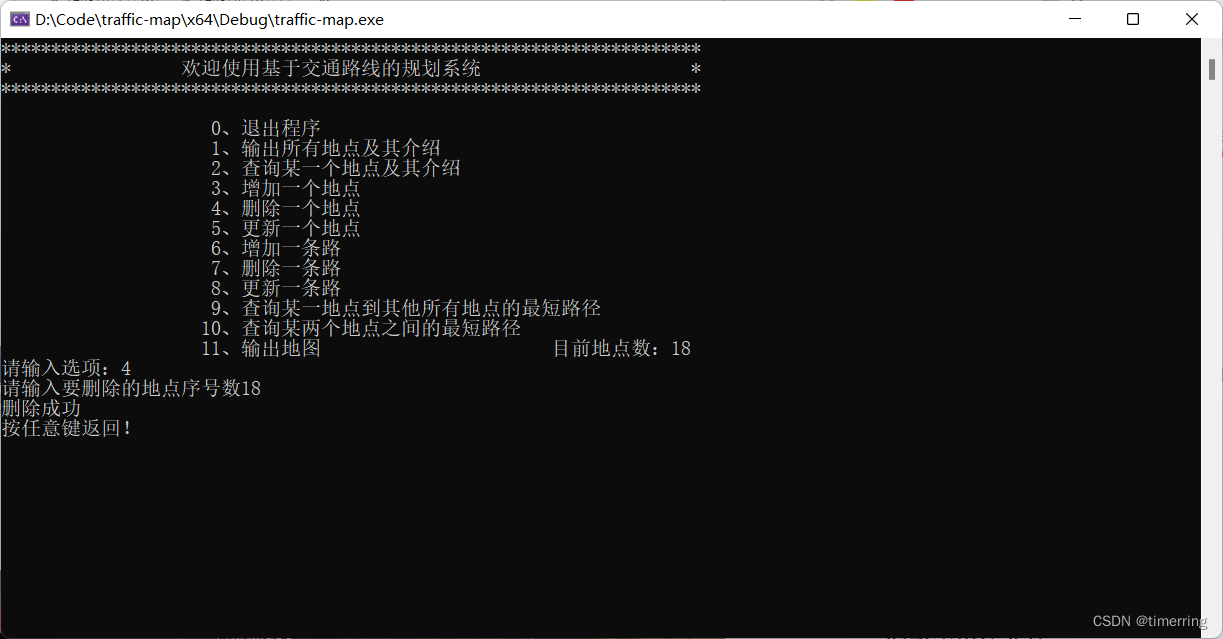5.更新一个地点，结果如下所示：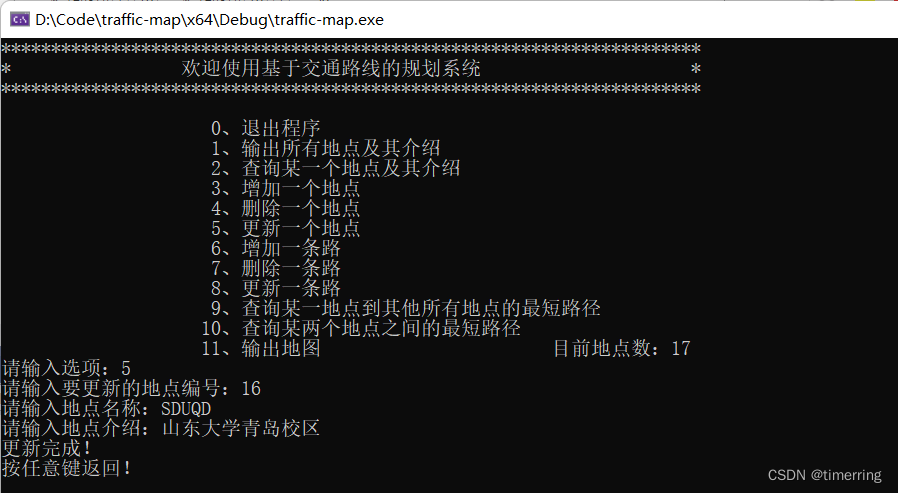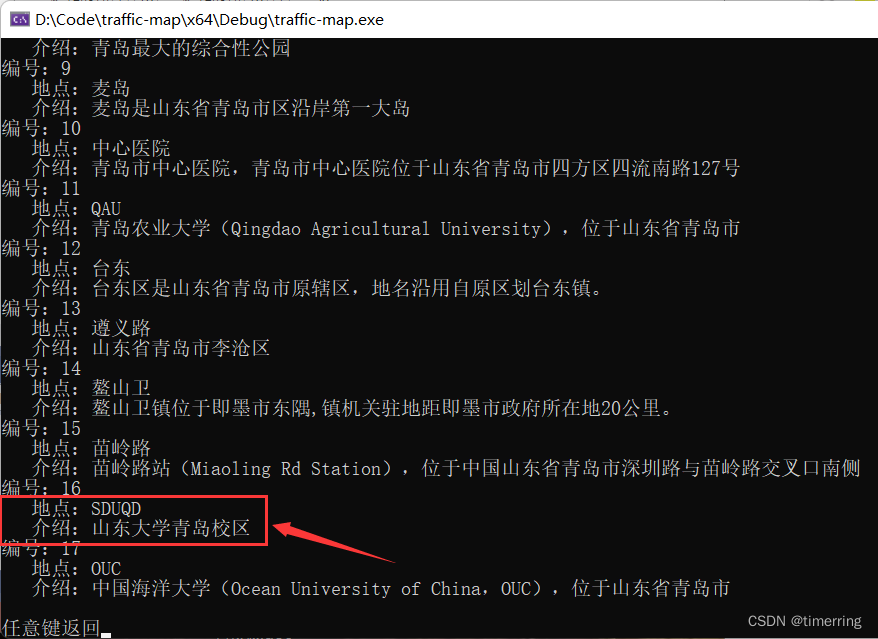6.增加一条路，结果如下所示：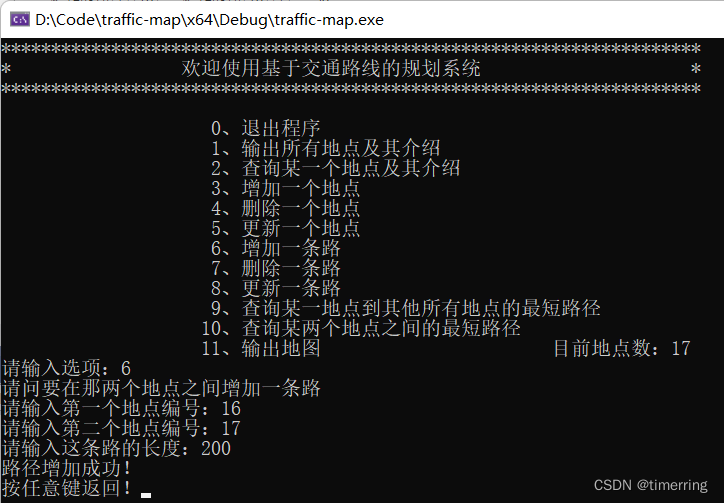7.删除一条路，结果如下所示：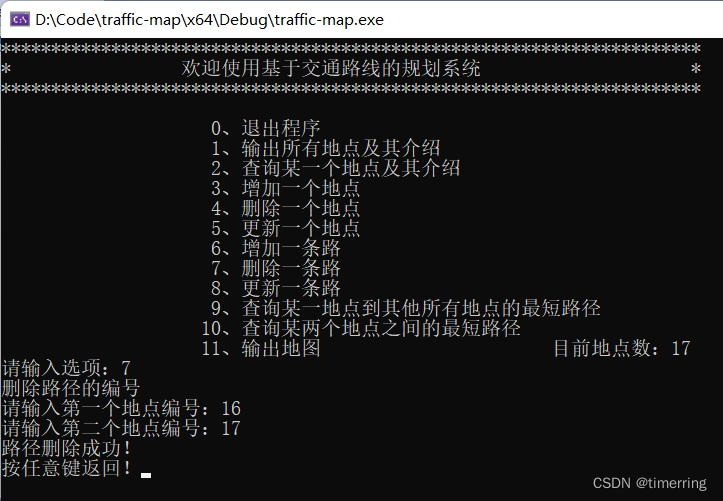8.更新一条路，结果如下所示：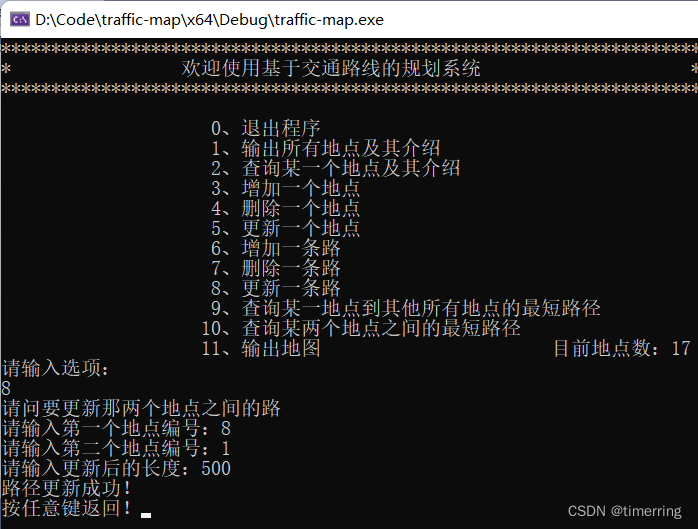9.查询某一地点到其他所有地点的最短路径，结果如下所示：

这里采用了Dijkstra算法，求解两点之间的最短路径，并且采用栈的方式输出该条路径。循环实现所有路径的输出。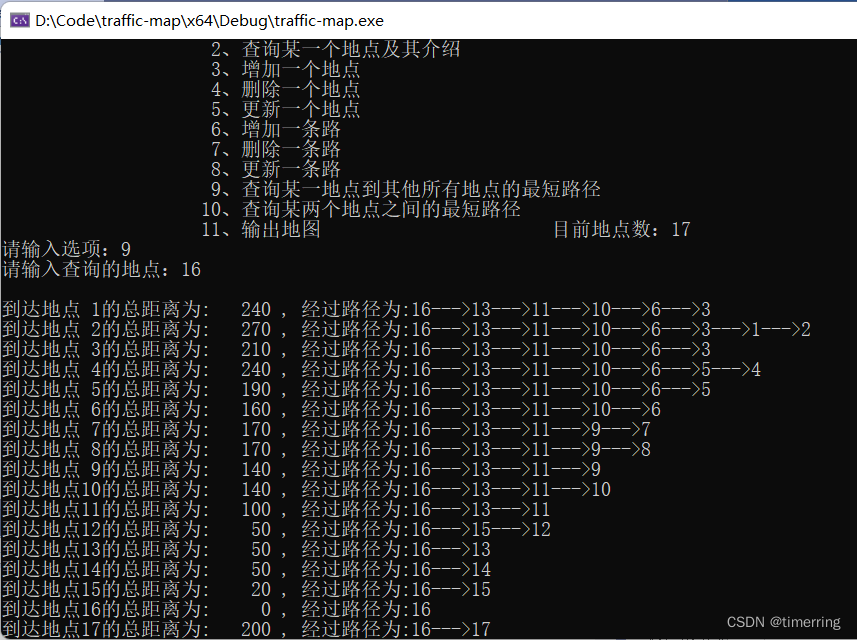10.查询某两个地点之间的最短路径，结果如下所示：

这里采用了Dijkstra算法，求解两点之间的最短路径，并且采用栈的方式输出该条路径。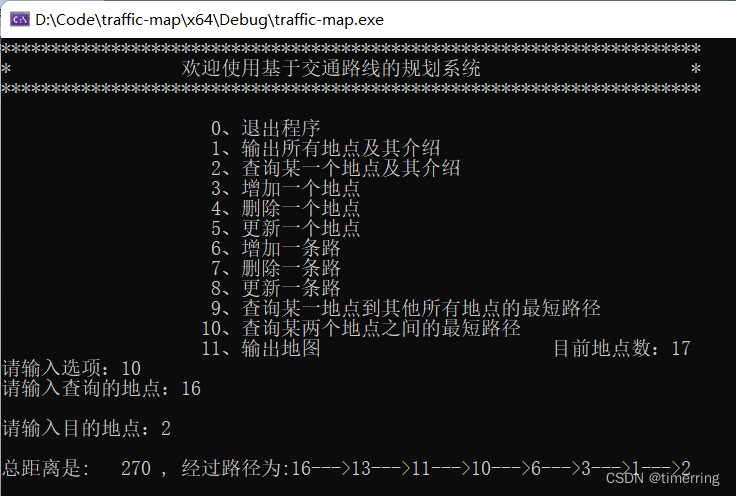11.输出地图，结果如下所示：

这里采用了graphics.h头文件库，针对地点的xy坐标属性，可视化无向图。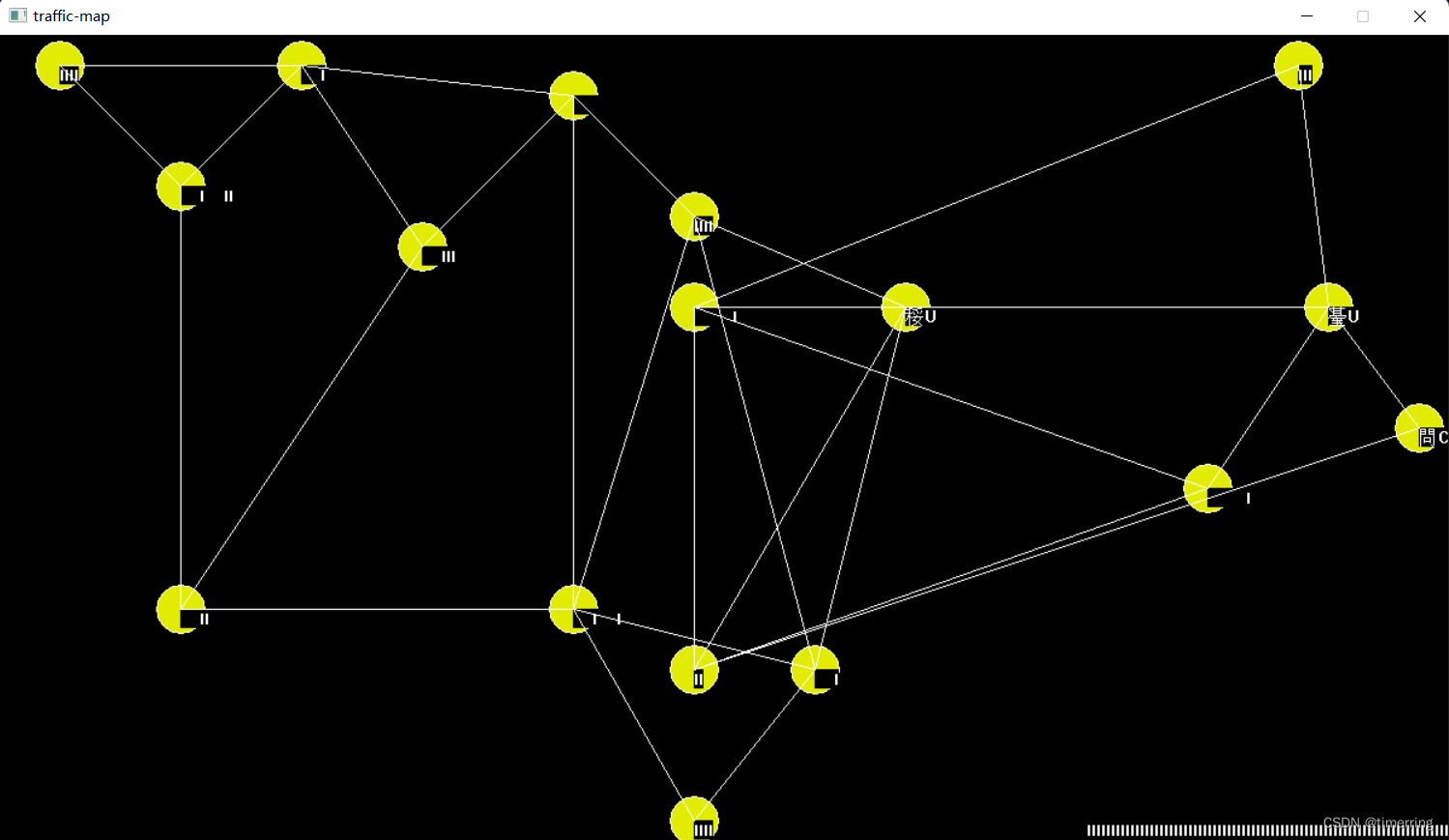# 七、总结心得

本课程设计是有关于基于交通路线的规划系统的实现，实验帮助我复习了有关于图的知识以及最短路径里的Dijkstra算法，在课设的选题方面上参考了一些优秀的思路，最后整个系统由我自行实现。

整个设计的核心思路是Dijkstra算法的实现，其基本思想是，设置顶点集合S并不断地作贪心选择来扩充这个集合。一个顶点属于集合S当且仅当从源到该顶点的最短路径长度已知。初始时，S中仅含有源。设u是G的某一个顶点，把从源到u且中间只经过S中顶点的路称为从源到u的特殊路径，并用数组dist记录当前每个顶点所对应的最短特殊路径长度。Dijkstra算法每次从V-S中取出具有最短特殊路长度的顶点u，将u添加到S中，同时对数组dist作必要的修改。一旦S包含了所有V中顶点，dist就记录了从源到所有其他顶点之间的最短路径长度。

此外，在路径输出功能函数上的设计，我采用了栈存储的方式，实现了路径溯源与倒序输出，较好的完成了路径的输出与追溯。对于增删改查功能的设计上采用了增删数组元素的基本思想，即将要删除的数组元素的右侧以及下侧的移动来实现节点的增删，路径的删除则是采用有关图的定义，将其路径重新设置为无穷。

有关于整个无向图的可视化部分，我采用了graphics.h图形库来实现，通过查阅了相关的博客学会了使用这个库，但是还是存在一些问题，这graphics.h图形库有关于outtextxy函数的重载的两个参数定义并不能很好地显示中文，关于这个问题还需要我进一步的探究。

此外，该课程设计还是存在许多不足，例如对于邻接矩阵实现存储的方式对于空间的复杂度要求过高，可以进一步采用邻接表、链表等存储结构，可以大大地降低空间复杂度。其次，在时间复杂度方面，可以很清晰地看到由于是线性查找，Dijkstra的时间复杂度是O（n2），效率并不高，我通过查阅文献的方式了解到可以进一步采用优先队列的思路优化它，在存储时就按照从小到大的顺序实现，这样在选择节点时直接取队首距离最小的节点即可，可以将时间复杂度优化到O（logn）左右。还有对于地点的设计，使用坐标xy只是用来graphics.h可视化的，并未考虑到实际距离的限制，这样可能会造成坐标差距很大，但是距离却很近等异常情况，因此可以通过计算坐标之间的欧氏距离，并将它设置为两点距离的最小值作为限制，防止异常情况的发生。

最后，整个课程设计的过程十分不易，从最初的选题，选定思路，函数模块设计，接口的对接，bug的调试耗费了很多心血，但是最终可以呈现出一个相对完整的项目还是令人喜悦的。

# 八、致谢&参考文献

1.graphics.h图形库用法总结

graphics.h图形库用法总结_i-Curve的博客-CSDN博客_graphics.h

2.graphic头文件函数_使用graphics.h来绘制图形

graphic头文件函数_使用graphics.h来绘制图形_weixin_39791653的博客-CSDN博客

3.Dijkstra算法应用(二) Emergency

Dijkstra算法应用(二) Emergency_Robbery07的博客-CSDN博客

4. 吴红波,王英杰,杨肖肖.基于Dijkstra算法优化的城市交通路径分析[J].北京交通大学学报,2019,43(04):116-121+130.

5.Visualization of shortest path

6. 基于最短路径的武汉地铁规划系统

https://github.com/wsxk/WuHan_Subway

7. HitWH_Map

GitHub - y0913011011/HitWH_Map: 哈工大威海地图系统（路径规划、信息查询）

展开全文数据结构 最短路径算法
• j++)//从 HT.data到HT[n].data匹配字符 { if (ch2[i] == HT[j].data || ch2[i] - 32 == HT[j].data)//当字符和HT[j].data相等 或 字符的ascii码-32转化为大写字母后HT[j].data相等时 { fstream f; f.open(...

首先需要三个txt文件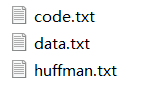huffman.txt文件存入各个节点的值，data.txt存入要编码的字符串，code.txt存放编码的结果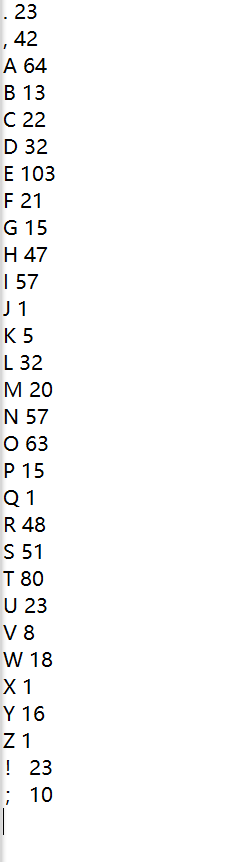如果出问题就是huffman.txt文件的最后一行不是空行，仔细看图中的光标位置code.txt为空

//zstu-wcj
#include<iostream>
#include<fstream>
#include<string>
using namespace std;
#define max 1000
typedef struct huffmantree//哈夫曼树结点
{
char data;//结点的值
int weight;//结点权值
int parent, lchild, rchild;//父节点，左右结点在数组中的位置下标
}HTNode, * HuffmanTree;
typedef char** HuffmanCode;
string chhh;//记录data.txt中的字符串
void Select(HuffmanTree& HT, int m, int& s1, int& s2)//在HT[k]中选择两个其双亲域为0且权值最小的结点,(1<=k<=i-1)
{                                                     // 并返回它们在HT中的序号s1和s2
int i, min1 = 10000, min2 = 10000; //先赋予最大值
for (i = 1; i <= m; i++)//找到未建树的最小值的下标
{
if (HT[i].weight < min1 && HT[i].parent == 0)  //当且仅当权值为最小值，且未构建到树中
{
min1 = HT[i].weight;
s1 = i;//记录下标
}
}

for (i = 1; i <= m; i++)//找到未建树的第二小的下标
{
if (HT[i].weight < min2 && HT[i].parent == 0 && i != s1)
{
min2 = HT[i].weight;
s2 = i;//记录下标
}
}
}
void Read_Data_To_Tree(HuffmanTree& HT, int n)
{
int m;
m = 2 * n - 1;//m = 所有结点的个数
for (int i = 0; i <= m; ++i) //将1~m号单元中的双亲、左孩子，右孩子的下标都初始化为0
{
HT[i].parent = 0;
HT[i].lchild = 0;
HT[i].rchild = 0;
}
ifstream myfile("huffman.txt");
HT.data = ' ';//找不到将空格从文件读入的方法
HT.weight = 186;//就先自己传了
int len;
for (len = 2; len <= n; ++len)//将huffman.txt的内容读到HT[]中
{
myfile >> HT[len].data >> HT[len].weight;
}

int s1, s2;
for (int i = n + 1; i <= m; ++i)
{
Select(HT, i - 1, s1, s2);

HT[s1].parent = i;
HT[s2].parent = i;
//得到新结点i，将s1和s2的父结点下标由0改为i
HT[i].lchild = s1;
HT[i].rchild = s2;                            //s1,s2分别作为i的左右孩子
HT[i].weight = HT[s1].weight + HT[s2].weight; //i 的权值为左右孩子权值之和
}
}

void Creat_HuffmanCode(HuffmanTree& HT, HuffmanCode* HC, int n)
{
int c, f, start;
*HC = new char* [n + 1];//*HC指向n个字符数组的第一个数组
char* cd = new char[n];//暂时存放哈夫曼编码
cd[n - 1] = '\0';
for (int i = 1; i <= n; i++)
{
start = n - 1;//猜测每个编码的长度不会超过所有叶子结点的个数之和
c = i;//先存储当前结点的下标
f = HT[i].parent;//父结点的下标
while (f != 0)//当HT[i]有父结点时，自底向上，寻找父结点，直到根结点为止
{
if (HT[f].lchild == c)//当父结点的左孩子==当前结点的下标时
cd[--start] = '0';//左0
else                             //因为是自底向上，所以是从cd的后往前填充编码
cd[--start] = '1';//右1
c = f;//c存储父结点的下标
f = HT[f].parent;//f为父结点的父结点的下标
}
(*HC)[i] = new char[n - start];//(*HC)[i]指向第i个字符串
strcpy_s((*HC)[i], n - start, &cd[start]);//将cd数组从start到末尾的部分复制到(*HC)[i]中
//该处为避免STL检查使用了strcpy
//但是第二个参数的设置没有仔细地验证
//也许存在差1问题
}
delete[] cd;
}
void data_to_code(HuffmanTree& HT, HuffmanCode* HC, int n)//将正文编码写入code.txt
{
string ch1, ch2;
bool flag = true;//使ch2第一次不加空格
ifstream infile("data.txt");
while (infile >> ch1)//每次都只能读到空格
{
if (flag == false)
{
ch2 += " ";
}
ch2 += ch1;
flag = false;
}
chhh = ch2;//data.txt中的完整字符串
infile.close();//将data.txt的内容存入字符串ch中
int num = ch2.size();

//清空文件内容
string dbfile = "code.txt";
ofstream fileout(dbfile.c_str(), ios::out | ios::trunc);
fileout.close();

for (int i = 0; i < num; i++)//遍历ch2字符串
{
for (int j = 1; j <= n; j++)//从 HT.data到HT[n].data匹配字符
{
if (ch2[i] == HT[j].data || ch2[i] - 32 == HT[j].data)//当字符和HT[j].data相等 或 字符的ascii码-32转化为大写字母后与HT[j].data相等时
{
fstream f;
f.open("code.txt", ios::out | ios::app);//文件追加输入方式
f << (*HC)[j];//将HT[j].data 对应的哈夫曼编码输入到文件中
f.close();
}
}
}
cout << "是否要打印各个字符对应的编码(1打印，2不打印)：" << endl;
int f = 100;
cin >> f;
if (f == 1)
{
for (int i = 1; i <= n; i++)
{
cout << HT[i].data << " " << (*HC)[i] << endl;
}
}
}

void decodingHuffmanCode(HuffmanTree HT, HuffmanCode* HC, bool* up, int n) {
int p = 2 * n - 1;//HT的最后一个节点是根节点，前n个节点是叶子节点
int i = 0;//指示测试串中的第i个字符
int j = 0;//指示结果串中的第j个字符
char result;//存储解码以后的字符串
string coding;//存储code.txt中的哈夫曼编码

ifstream infile("code.txt");
infile >> coding;//将code.txt中的二进制字符串输入到coding
infile.close();

int num = coding.size();//二进制编码长度
while (i < num)//依次读取二进制编码
{
if (coding[i] == '0')  p = HT[p].lchild;//若读到的编码为1，则p跳转到左孩子结点
if (coding[i] == '1')  p = HT[p].rchild;//若读到的编码为0，则p跳转到左孩子结点

if (p <= n)           //p<=n则表明p为叶子节点,因为在构造哈夫曼树HT时，HT的m个节点中前n个节点为叶子节点
{
result[j] = HT[p].data;
j++;
p = 2 * n - 1;//p重新指向根节点
}
i++;
}
result[j] = '\0';//结果串的结束符
//因为 HT[p].data中的数据都为大写字母
//所以result数组中全为大写字母
//下面的for循环通过up数组的判定进行转换
for (int c = 0; c <= j; c++)
{
if (up[c] == 1)//非小写字母时直接输出
{
cout << result[c];
}
else//小写字母时将result的ascii码+32转化为小写字母
{
char down = result[c] + 32;

cout << down;
}
}
}
int main()
{
HuffmanCode HC;
HuffmanTree HT;

fstream fin("huffman.txt", ios::in);
char c;
int line = 0;
while (fin.get(c))//统计data.txt文件的行数，行数line+1即为结点数
{
if (c == '\n')//读到换行符时累加
line++;
}
fin.close();
int lenth = 0;
int n = line + 1;//行数
int m = 2 * n - 1;//结点数
HT = new HTNode[m + 1];  //不用HTNode,所以分配m+1个单元，HT[m] 表示根结点

cout << "************哈夫曼编译码***********" << endl;
cout << "*********1.读取数据并建树**********" << endl;
cout << "*********2.编码          **********" << endl;
cout << "*********3.解码并打印    **********" << endl;
cout << "*********4.打印各个编码  **********" << endl;
cout << "***********************************" << endl;
int choice = -1;
while (choice != 0)
{
cout << "请输入：";
cin >> choice;
switch (choice)
{
case 1:
Creat_HuffmanCode(HT, &HC, n);//根据HT中的权值建树
cout << "建立成功" << endl;
break;
case 2:
data_to_code(HT, &HC, n);//将正文编码写入code.txt
cout << "编码成功" << endl;
break;
case 3:
bool up;//字符串中每个字符对应的flag，用来判断是否为大写字母
lenth = chhh.size();//chhh是从data.txt中读到的字符串

for (int i = 0; i < lenth; i++)//
{
up[i] = true;                   //默认每个字符都是大写
if (chhh[i] > 90) up[i] = false;//当字符的ascii码值＞90时设为false   （Z的ascii码为90）
}
cout << "解码为：" << endl;
decodingHuffmanCode(HT, &HC, up, n);//解码
cout << endl;
break;
}
}

return 0;
}


有不明白的地方可以加群讨论（没错只有我一个人）484266833

展开全文数据结构
• 数据结构与算法课设——医院候诊管理系统.docx《数据结构与算法课设——医院候诊管理系统.docx《数据结构与算法课设——医院候诊管理系统.docx《数据结构与算法课设——医院候诊管理系统.docx《数据结构与...
• 本文主要介绍了数据结构与算法课程设计专栏的更新内容。 今天是2022年的第一天，首先先和各位读者道一声新年快乐！新的一年预祝各位平安顺利。让我们一起向未来！同时祝福祖国，国泰民安！！！ 我呐，也是刚刚经历...数据结构
• 数据结构与算法课设——医院候诊管理系统.pdf《数据结构与算法课设——医院候诊管理系统.pdf《数据结构与算法课设——医院候诊管理系统.pdf《数据结构与算法课设——医院候诊管理系统.pdf《数据结构与算法...
• 《(桂电)学生成绩管理系统(论文加源代码) 数据结构与算法课设》由会员分享，可在线阅读，更多相关《(桂电)学生成绩管理系统(论文加源代码) 数据结构与算法课设(6页珍藏版)》请在人人文库网上搜索。1、桂电)学生成绩...
• 四川大学计算机学院-数据结构与算法课程设计-高分报告-公园导游系统.doc 都是自己非常认真完成的，每一个要点都实现到位，还额外实现了创新内容。 最后得到的分数也很好
• 数组（Array）是一种线性表数据结构。它用一组连续的内存空间，来存储一组具有相同类型的数据。 第一是线性表（Linear List）。 而它相对立的概念是非线性表，比如二叉树、堆、图等。 第二个是连续的内存空间和...数据结构
• 跳马问题。要求在64个国际象棋格子,任意位置放一个马,如何不重复地把格子走完。
• 数据结构与算法课设——医院候诊管理系统.doc数据结构
• 算法与数据课设参考题目,一、职工信息的综合运算 有一个职工文件emp.txt,职工记录包含职工编号、姓名、部门号和工资数，设计一个程序实现以下功能 1）从emp.txt文件中读出职工记录，建立一个带头节点的单链表L 2）...
• [基本要求] 直接插入排序、希尔排序、直接选择排序、堆排序、起泡排序、...（2）比较各种排序算法对同一组数据排序所需要的关键字比较次数和关键字移动次数，至少使用5组数据进行比较； （3）对比较结果进行简单分析。
• 数据结构课程设计最小生成树的普里姆算法课设.doc
•数据结构
• 实用文案 目 录 引言 1 系统概述 1.1 系统功能 1.2 系统作用和特点 2 需求分析 2.1 功能要求 2.2 性能要求 2.3 数据需求 2.4 开发环境 3 详细设计 3.1 登陆界面主界面的实现 3.2 详细设计思想 3.3 系统结构体的...
• 排序数据随机产生，针对随机案例，对冒泡排序、箱子排序、堆排序、归并算法，提供排序执行过程的动态图形演示。...

# 数据结构与算法课设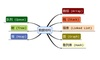数据结构 订阅